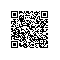# 使用python制作时间戳转换工具

python 时间戳转日期 日期转时间戳

1.每次打开过程步骤繁琐，即使收藏了也要先打开浏览器，然后点击
2.如果打开的浏览器标签足够多的话，根本找不到（就是说的我这样的人）
3.等

## 本文的环境

• python 3.6
• time 库
• tkinter 库


from tkinter import *
from time import *

'''
1、这个程序实现时间戳和日期格式的相互转换。
2、使用grid方法按照表格方式对组件位置进行安排
3、通过Button按钮进行转换和刷新操作。
4、通过Entry来获取用户输入。
'''
root = Tk()
root.title('时间戳转换')
root.resizable(0,0)#禁止拉伸 会变丑
# 对变量进行创建，和数据初始化
Label1 = Label(root, text='时间戳:').grid(row=0, column=0)
Label2 = Label(root, text='日期:').grid(row=1, column=0)
v1 = StringVar()
p1 = StringVar()
v1.set(int(time()))

Label3 = Label(root, text='日期:').grid(row=3, column=0)
Label4 = Label(root, text='时间戳').grid(row=4, column=0)
v2 = StringVar()
p2 = StringVar()
timeArray1 = localtime(int(time()))
v2.set(strftime("%Y-%m-%d %H:%M:%S", timeArray1))
p2.set(int(time()))
#时间戳转换成日期
def trans1():

e1 = Entry(root, textvariable=v1)  # Entry 是 Tkinter 用来接收字符串等输入的控件.
e2 = Entry(root, textvariable=p1)

timeArray = localtime(int(e1.get()))
p1.set(strftime("%Y-%m-%d %H:%M:%S", timeArray))
#日期转换为时间戳
def trans2():
e3 = Entry(root, textvariable=v2)  # Entry 是 Tkinter 用来接收字符串等输入的控件.
e4 = Entry(root, textvariable=p2)
p2.set(int(mktime(strptime(e3.get(), "%Y-%m-%d %H:%M:%S"))))
#刷新第二个模组
def refresh():
timeArray1 = localtime(int(time()))
v2.set(strftime("%Y-%m-%d %H:%M:%S", timeArray1))
p2.set(int(time()))

Button(root, text='转换', width=10, command=trans1) \
Button(root, text='转换', width=10, command=trans2) \
Button(root, text='刷新', width=10, command=refresh) \
Button(root, text='退出', width=10, command=root.quit) \
trans1()
trans2()
#设置窗口初始显示位置
sw = root.winfo_screenwidth()
sh = root.winfo_screenheight()
x = (sw) / 2
y = (sh) / 2
root.geometry("+%d+%d" %(x,y))
mainloop()



## pyinstaller的安装和使用

### 安装

pip install pyinsatller

#安装直接运行一下如下命令
pyinstaller
#如果能运行会提示选项

### 打包文件

#打包的命令（在命令行中运行，如果不成功记得配置好环境变量）
pyinsatller -F -w D:\python\timeTran.py
#选项介绍
#-F –onefile    产生一个文件用于部署 (参见XXXXX).
#-w,–windowed,–noconsole 使用Windows子系统执行.当程序启动的时候不会打开命令行(只对Windows有效) 就是不会显示一个黑窗口（太丑了，还要手动去关闭）如果不知道什么意思，可以自己去试试

github地址：https://github.com/VinterHe/timeTransverter 里面有打包好的exe文件可以直接拿去用使用钉钉扫一扫加入圈子
+ 订阅